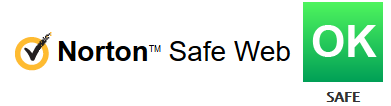# Multiplication Tables sheet

## Enregistrer, Remplir les champs vides, Imprimer, Terminer!Learn how to multiply! How to work with a Multiplication Tables overview? Download this Multiplication Table template that will perfectly suit your needs!

Formats de fichiers gratuits disponibles:

.docx

• Ce document a été certifié par un professionnel
• 100% personnalisable

Évaluation du modèle: 7

Aucun Malware/Virus trouvé, scanné par:Education Éducation math mathematics mathématiques multiply multiplier Multiplication learn how to multiply apprendre à se multiplier multiplication table table de multiplication multiplication sheet feuille de multiplication sample multiplication multiplication d'échantillon multiplication tables tables de multiplication printable multiplication table multiplication table printable multiplication table chart 12 multiplication table multiplication table up to 100 multiplication table up to printable multiplication chart memorize multiplication tables multiplication table 12 multiplication tables to 20

Learn how to multiply! How to work with a Multiplication Tables overview? Download this Multiplication Table template that will perfectly suit your needs!

In math, the multiplication table is a a common mathematical table that is useful to memorize the tables, often from 1 to 12 for children. It defines the multiplication operation for an algebraic system.

Mathematicians regularly need spreadsheets, worksheets or forms, and often more than any other industry. Therefore, we support you by providing this Multiplication Table template, which will save your time, cost and efforts and help you to reach the next level of success in your studies, work or business!

This blank Multiplication Table is intuitive, ready-to-use and structured in a smart way. Try it now and let this mathematics template inspire you. We certainly encourage to use this Multiplication Table for your own good and are confident it will fit your needs

Our site is often updated with new educational and math templates.

AVERTISSEMENT
Rien sur ce site ne doit être considéré comme un avis juridique et aucune relation avocat-client n'est établie.

Si vous avez des questions ou des commentaires, n'hésitez pas à les poster ci-dessous.Voir plus